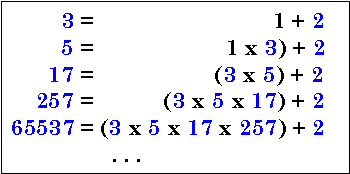# It Must Be A Prime Number!In mathematics, a Fermat Number is a positive integer of the form $F_n = 2^{\left (2^n \right)} + 1$

Given that $F_7 = 340282366920938463463374607431768211457,$
what are the last three digits of the smallest prime factor of $F_7$?

×